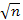## Statistical Inference - Simulation

We will first use a new applet to model the randomness used in this study, assuming there is no effect from chip type, to see how surprising it is to find a mean difference at least as extreme as the one you just determined when the times are truly interchangeable. To do this, we will assume it doesn't matter which time was in the %chip1type% column and which time was in the %chip2type%column. So we will assign the times for each person to the two columns by just flipping a coin. If the coin flip is heads, we will swap the values. If the coin flip is tails, the values stay put.

(f) Describe the distribution of the Average Difference variable from different randomizations (shape, center, variability). Explain why we expect the mean of this distribution to be close to zero.

(g) Report the approximate p-value and include a one-sentence interpretation of the p-value in context.

Again, we can consider a mathematical model for the simulated null distribution of the standardized statistics.

• Use the radio button above the null distribution to select the t-statistic. Now check the box to Overlay t distribution.

(h) Does the t-distribution appear to predict the behavior of the null distribution of the t-statistic reasonably well?

(i) Use the sample data (and your null hypothesis) to calculate the observed t-statistic for these differences:
(observed statistic - hypothesized parameter value)/(sd/).

(j) Now enter this value in the Count Samples box. Determine the theory-based p-value and compare it to your p-value from the simulation. (Include a screen capture of the p-value output.)

(k) With the theory-based approach we can also get a confidence interval. Check the Compute 95% CI for mean difference box on the far left (under the Data window). Report and interpret the resulting interval. [Hint: Be careful in the wording of your parameter!]